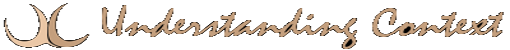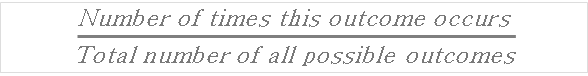Anatomy of Insight: Prediction and Qualitative BI

## Theoretical Probability Formula

By Joe Roushar | Published May 23, 2018 | Full size is 588 × 76 pixelsEmpirical Probability Formula Random Probability

Theoretical Probability Formula

Theoretical Probability Formula

This site uses Akismet to reduce spam. Learn how your comment data is processed.